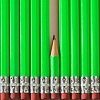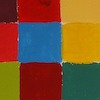# Angles, Polygons and Geometrical Proof - Stage 4### Triangle Midpoints

##### Age 14 to 16Challenge Level

You are only given the three midpoints of the sides of a triangle. How can you construct the original triangle?##### Age 14 to 16Challenge Level

Two ladders are propped up against facing walls. The end of the first ladder is 10 metres above the foot of the first wall. The end of the second ladder is 5 metres above the foot of the second wall. At what height do the ladders cross?### Sitting Pretty

##### Age 14 to 16Challenge Level

A circle of radius r touches two sides of a right angled triangle, sides x and y, and has its centre on the hypotenuse. Can you prove the formula linking x, y and r?### Napkin

##### Age 14 to 16Challenge Level

A napkin is folded so that a corner coincides with the midpoint of an opposite edge . Investigate the three triangles formed .### Angle Trisection

##### Age 14 to 16Challenge Level

It is impossible to trisect an angle using only ruler and compasses but it can be done using a carpenter's square.### Squirty

##### Age 14 to 16Challenge Level

Using a ruler, pencil and compasses only, it is possible to construct a square inside any triangle so that all four vertices touch the sides of the triangle.### Trapezium Four

##### Age 14 to 16Challenge Level

The diagonals of a trapezium divide it into four parts. Can you create a trapezium where three of those parts are equal in area?### Nicely Similar

##### Age 14 to 16Challenge Level

If the hypotenuse (base) length is 100cm and if an extra line splits the base into 36cm and 64cm parts, what were the side lengths for the original right-angled triangle?### Partly Circles

##### Age 14 to 16Challenge Level

What is the same and what is different about these circle questions? What connections can you make?### Making Sixty

##### Age 14 to 16Challenge Level

Why does this fold create an angle of sixty degrees?##### Age 14 to 16Challenge Level

Explore when it is possible to construct a circle which just touches all four sides of a quadrilateral.##### Age 11 to 16Challenge Level

Draw some quadrilaterals on a 9-point circle and work out the angles. Is there a theorem?### Kite in a Square

##### Age 14 to 18Challenge Level

Can you make sense of the three methods to work out what fraction of the total area is shaded?##### Age 14 to 18Challenge Level### Tied Up

##### Age 14 to 16 ShortChallenge Level

How much of the field can the animals graze?### Angles, Polygons and Geometrical Proof Short Problems

##### Age 11 to 16

A collection of short problems on Angles, Polygons and Geometrical Proof.### Same Length

##### Age 11 to 16Challenge Level

Construct two equilateral triangles on a straight line. There are two lengths that look the same - can you prove it?### Isosceles Seven

##### Age 14 to 16Challenge Level

Is it possible to find the angles in this rather special isosceles triangle?### The Square Under the Hypotenuse

##### Age 14 to 16Challenge Level

Can you work out the side length of a square that just touches the hypotenuse of a right angled triangle?### Overlapping Annuli

##### Age 14 to 16 ShortChallenge Level

Just from the diagram, can you work out the radius of the smaller circles?### Circled Square

##### Age 14 to 16 ShortChallenge Level

Can you find the area of this square inside a circle?### Overlapping Semicircles

##### Age 14 to 16 ShortChallenge Level

Two semicircles overlap, can you find this length?### Octagonal Ratio

##### Age 14 to 16 ShortChallenge Level

Can you find the ratio of the area shaded in this regular octagon to the unshaded area?### Block Challenge

##### Age 14 to 16 ShortChallenge Level

Can you work out the shaded area in this shape?### Height and Sides

##### Age 14 to 16 ShortChallenge Level

Can you find the area of the triangle from its height and two sides?### Circular Inscription

##### Age 14 to 16 ShortChallenge Level

In the diagram, the radius of the circle is equal to the length AB. Can you find the size of angle ACB?### 3-4-5 Circle

##### Age 14 to 16 ShortChallenge Level

Can you find the radius of the circle inscribed inside a '3-4-5 triangle'?### Slide Height

##### Age 14 to 16 ShortChallenge Level

How high is the top of the slide?### Triangular Intersection

##### Age 14 to 16 ShortChallenge Level

What is the largest number of intersection points that a triangle and a quadrilateral can have?### Two Equilateral Triangles

##### Age 14 to 16 ShortChallenge Level

Prove that these two lengths are equal.### Semicircle in a Triangle

##### Age 14 to 16 ShortChallenge Level

A semicircle is drawn inside a right-angled triangle. Find the distance marked on the diagram.### Folded Square

##### Age 14 to 16 ShortChallenge Level

This square piece of paper has been folded and creased. Where does the crease meet the side AD?### Tunnel Vision

##### Age 14 to 16 ShortChallenge Level

How wide is this tunnel?### Square and Triangle

##### Age 14 to 16 ShortChallenge Level

Can you find the area of the yellow square?### Doubly Isosceles

##### Age 14 to 16 ShortChallenge Level

Find the missing distance in this diagram with two isosceles triangles### Circles on a Triangle

##### Age 14 to 16 ShortChallenge Level

Find the missing length on this diagram.### Cat on a Wall

##### Age 14 to 16 ShortChallenge Level

How high is the wall that this cat is lying on?